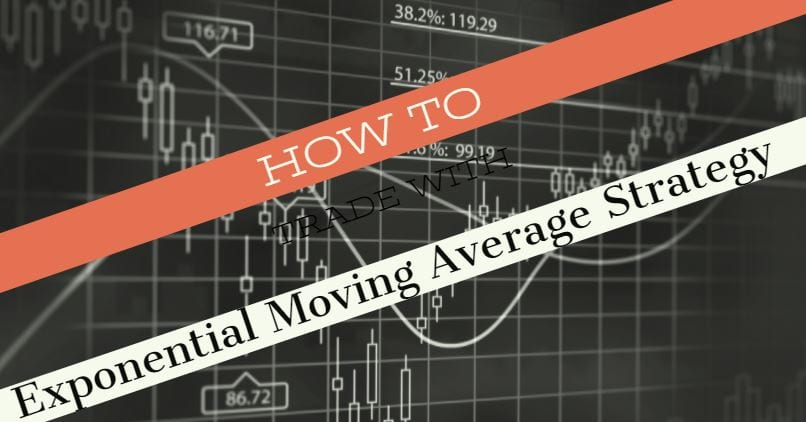The exponential moving average is the oldest form of technical analysis. It is one of the most popular trading indicators used by thousands of traders. In this step-by-step guide, you’ll learn a simple exponential moving average strategy. Use what you learn to turn your trading around and become a successful, long-term trader! A moving average can be a very effective indicator. Many traders use exponential moving averages, an effective type of moving average indicator, to trade in a variety of markets.

An exponential moving average strategy, or EMA strategy, is used to identify the predominant trend in the market. It can also provide the support and resistance level to execute your trade.

Our team at Trading Strategy Guides has already covered the topic, trend following systems. You can review the trend here, MACD Trend Following Strategy - Simple to Learn Trading Strategy. You can also learn the basics of support and resistance here, Support and Resistance Zones – Road to Successful Trading.

Make sure you go through the recommended articles if you want to better understand how the market works. Building a foundation of understanding will help you dramatically improve your outcomes as a trader.

The Exponential Moving Average EMA Strategy is a universal trading strategy that works in all markets. This includes stocks, indices, Forex, currencies, and the crypto-currencies market, like the virtual currency Bitcoin. If the exponential moving average strategy works on any type of market, they work for any time frame. In simple terms, you can trade with it on your preferred chart. Also, read the hidden secrets of moving average.

Let’s first examine what a moving average is and the exponential moving average formula. After, we will dive into some of the key rules of the exponential moving average strategy,

## Exponential Moving Average Formula and Exponential Moving Average Explained

The exponential moving average is a line on the price chart that uses a mathematical formula to smooth out the price action. It shows the average price over a certain period of time. The EMA formula puts more weight on the recent price. This means it’s more reliable because it reacts faster to the latest changes in price data.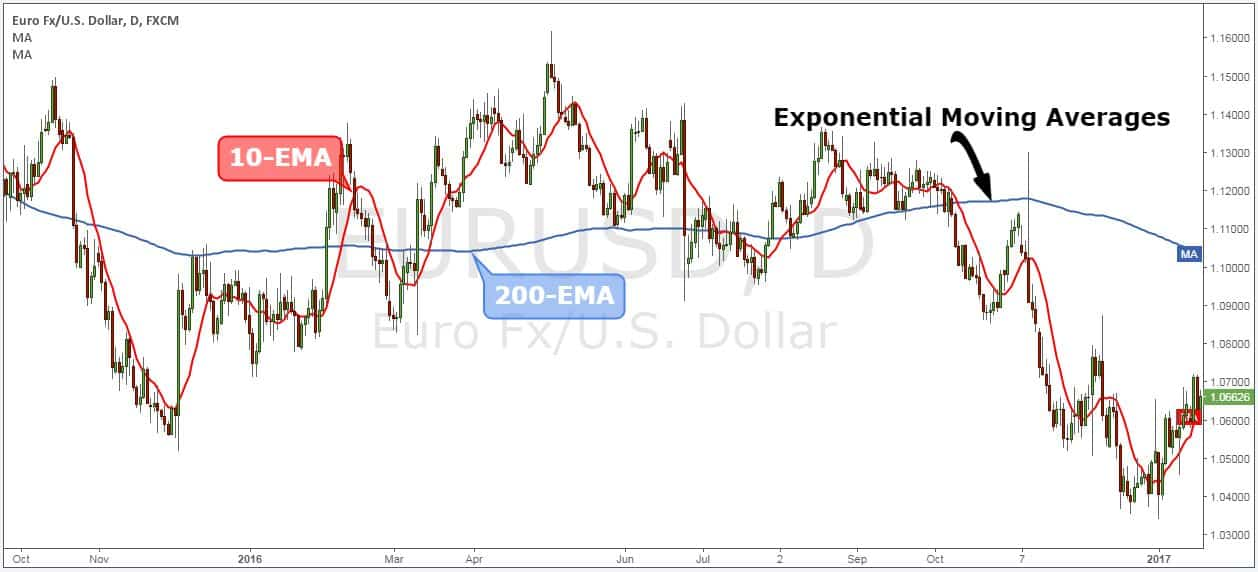An exponential moving average tries to reduce confusion and noise of everyday price action. Second, the moving average smooths the price and reveals the trend. It even sometimes reveals patterns that you can't see. The average is also more reliable and accurate in forecasting future changes in the market price.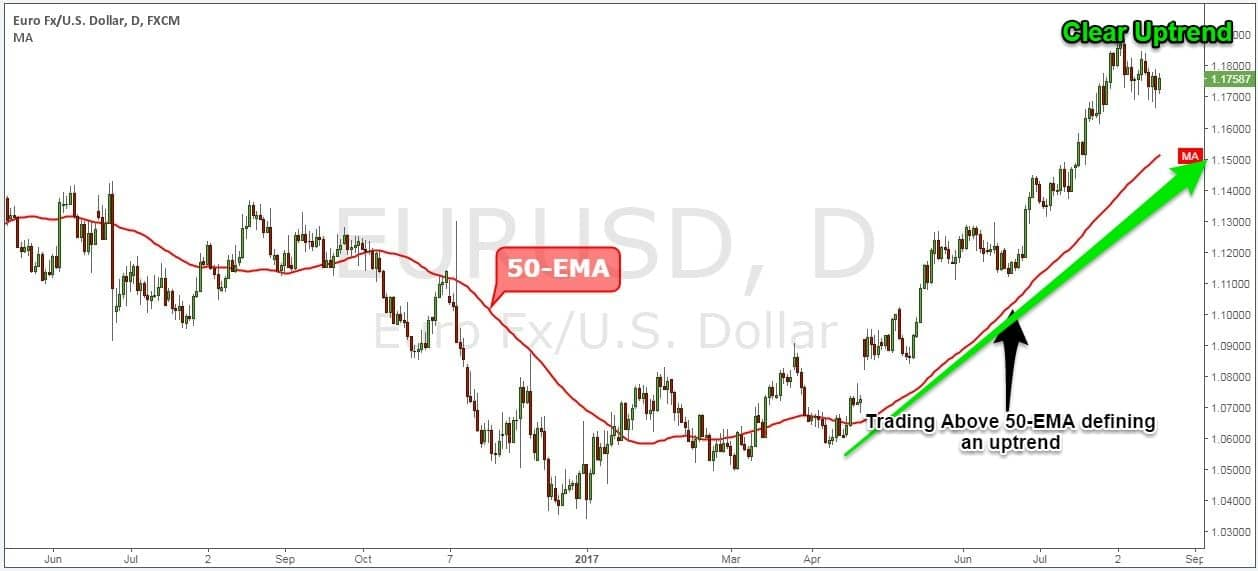There are 3 steps for the exponential moving average formula and calculating the EMA. The formula uses a simple moving average SMA as the starting point for the EMA value. To calculate the SMA, take the sum of the number of time periods and divide by 20.

We need a multiplier that makes the moving average put more focus on the most recent price.

The moving average formula brings all these values together. They make up the moving average.

The exponential moving average formula below is for a 20-day EMA:

##### EMA = {Close - EMA(previous day)} x multiplier + EMA(previous day).

The general rule is that if the price trades above the moving average, we’re in an uptrend. As long as we stay above the exponential moving average, we should expect higher prices. Conversely, if we’re trading below, we’re in a downtrend. As long as we trade below the moving average, we should expect lower prices.Before we go any further, we always recommend writing down the trading rules on a piece of paper. This exercise will step up your learning curve and you’ll become a better trader.

Let’s get started…

### Exponential Moving Average Strategy

Our exponential moving average strategy is comprised of two elements. The first degree to capture a new trend is to use two exponential moving averages as an entry filter.

By using one moving average with a longer period and one with a shorter period, we automate the strategy. This removes any form of subjectivity from our trading process.

#### Step #1: Plot on your chart the 20 and 50 EMA

The first step is to properly set up our charts with the right moving averages. We can identify the EMA crossover at the later stage. The exponential moving average strategy uses the 20 and 50 periods EMA.

Most standard trading platforms come with default moving average indicators. It should not be a problem to locate the EMA either on your MT4 platform or Tradingview.Now, we’re set to go a look more closely to the price structure. This brings us to the next step of the strategy.

#### Step #2: Wait for the EMA crossover and for the price to trade above the 20 and 50 EMA.

The second rule of this moving average strategy is the need for the price to trade above both 20 and 50 EMA. Secondly, we need to wait for the EMA crossover, which will add weight to the bullish case.

We refer to the EMA crossover for a buy trade when the 50-EMA crosses above the 50-EMA.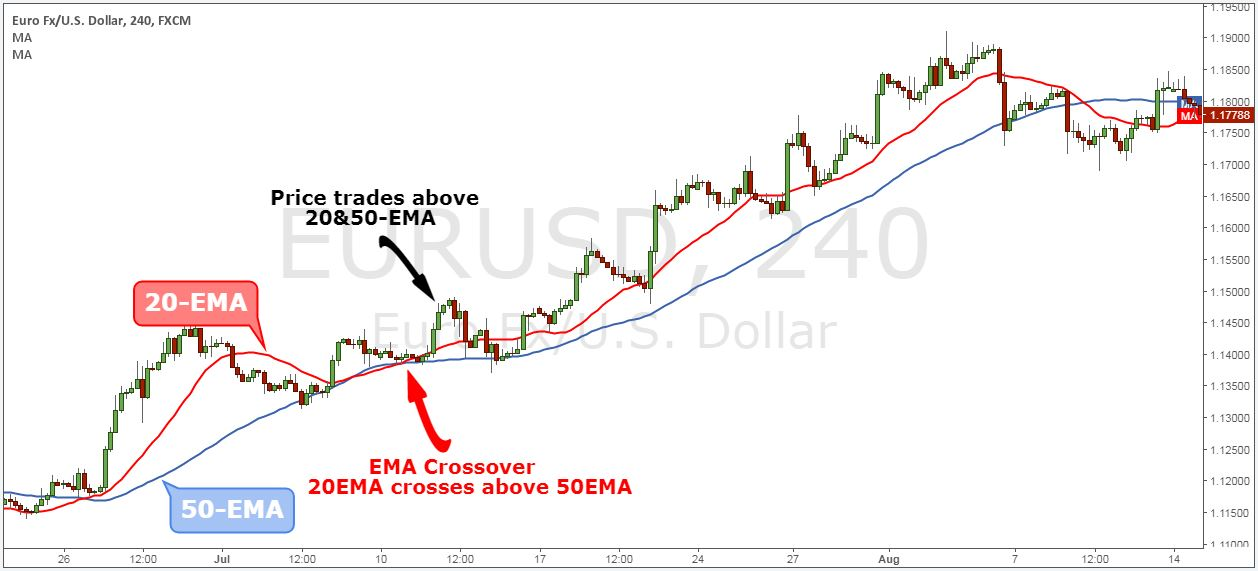By looking at the EMA crossover, we create an automatic buy and sell signals.

Since the market is prone to false breakouts, we need more evidence than a simple EMA crossover. At this stage, we don’t know if the bullish sentiment is strong enough to push the price further after we buy to make a profit.

To avoid the false breakout, we added a new confluence to support our view. This brings us to the next step of the strategy.

#### Step #3: Wait for the zone between 20 and 50 EMA to be tested at least twice, then look for buying opportunities.

The conviction behind this moving average strategy relies on multiple factors. After the EMA crossover happened, we need to exercise more patience. We will wait for two successive and successful retests of the zone between the 20 and 50 EMA.

The two successful retests of the zone between 20 and 50 EMA give the market enough time to develop a trend.

Never forget that no price is too high to buy in trading. And no price is too low to sell.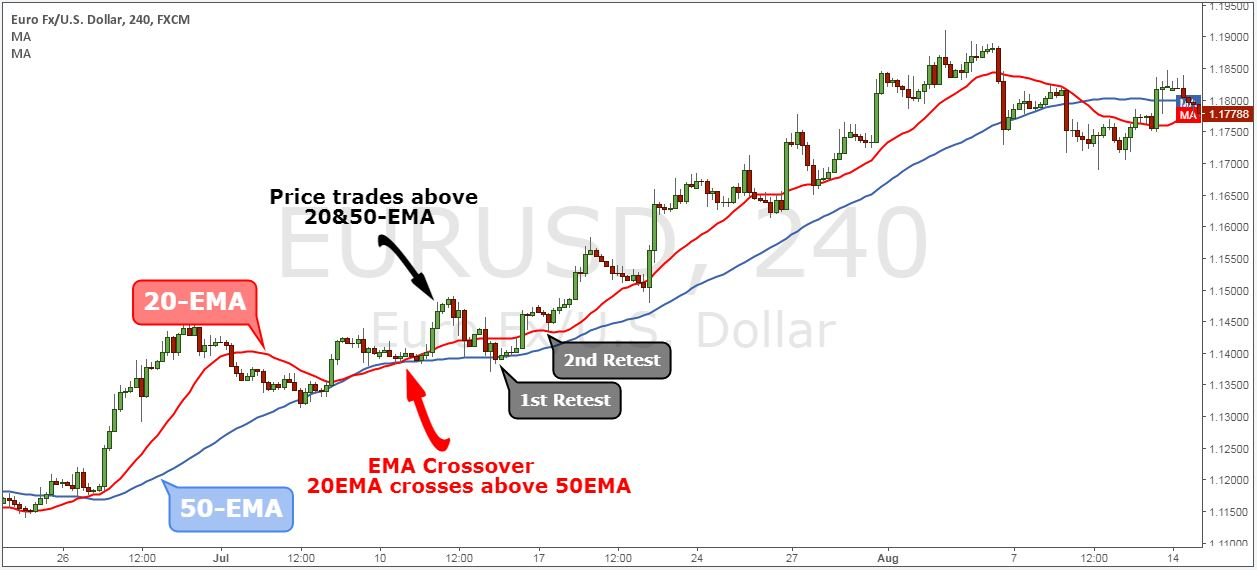Note* When we refer to the “zone between 20 and 50EMA,” we actually don’t mean that the price needs to trade in the space between the two moving averages.

We just wanted to cover the whole price spectrum between the two EMAs. This is because the price will only briefly touch the shorter moving average (20-EMA). But this is still a successful retest.

Now, we still need to define where exactly we are going to buy. This brings us to the next step of the strategy.

#### Step #4: Buy at the market when we retest the zone between 20 and 50 EMA for the third time.

If the price successfully retests the zone between 20 and 50 EMA for the third time, we go ahead and buy at the market price. We now have enough evidence that the bullish momentum is strong to continue pushing this market higher.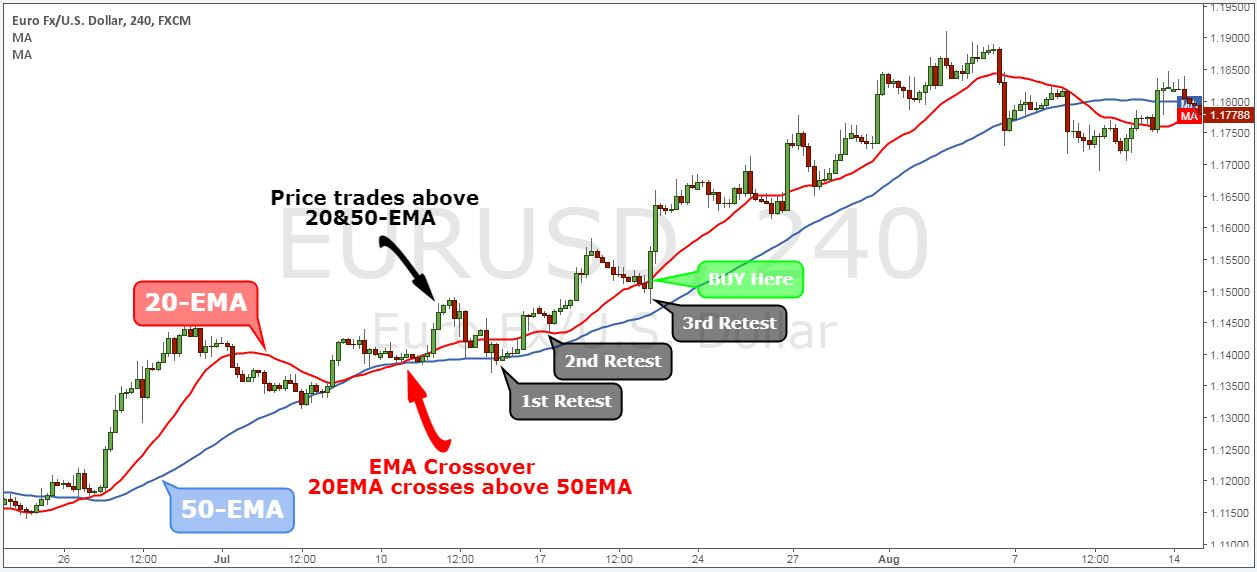Now, we still need to define where to place our protective stop loss and where to take profits. This brings us to the next step of the strategy.

#### Step #5: Place the protective Stop Los 20 pips below the 50 EMA

After the EMA crossover happened, and after we had two successive retests, we know the trend is up. As long as we trade above both exponential moving averages the trend remains intact.

In this regard, we place our protective stop loss 20 pips below the 50 EMA. We added a buffer of 20 pips because we understand we’re not living in a perfect world. The market is prone to do false breakouts.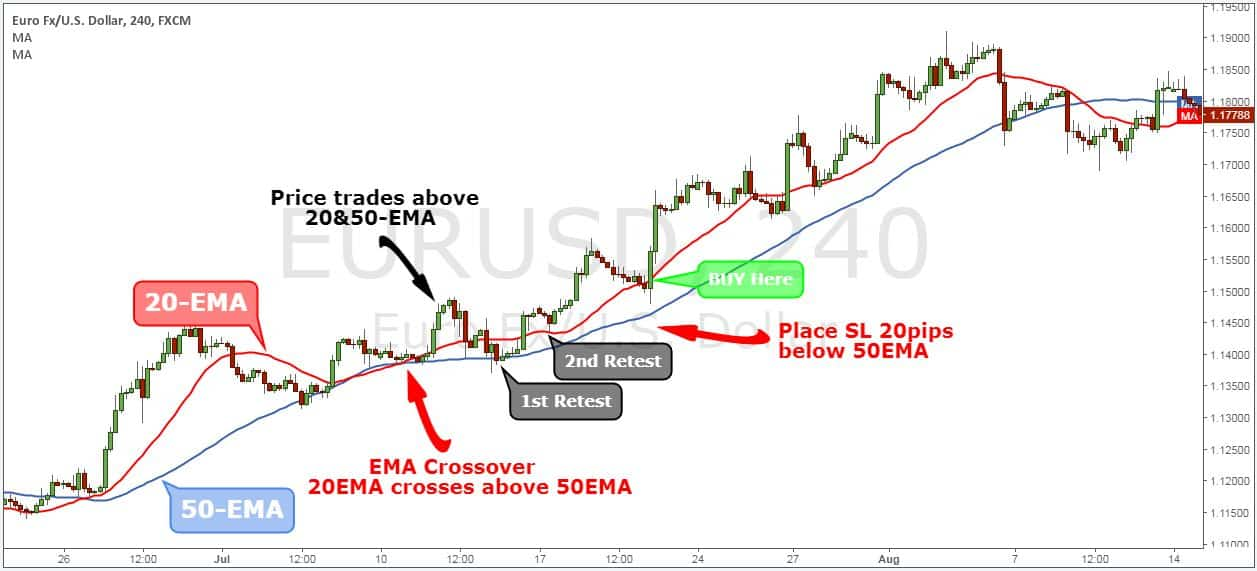The last part of our EMA strategy is the exit strategy. It is based again on the exponential moving average.

#### Step #6: Take Profit once we break and close below the 50-EMA

In this particular case, we don't use the same exit technique as our entry technique, which was based on the EMA crossover.

If we waited for the EMA crossover to happen on the other side, we would have given back some of the potential profits. We need to consider the fact that the exponential moving averages are a lagging indicator.

The exponential moving average formula used to plot our EMAs allow us to still take profits right at the time the market is about to reverse.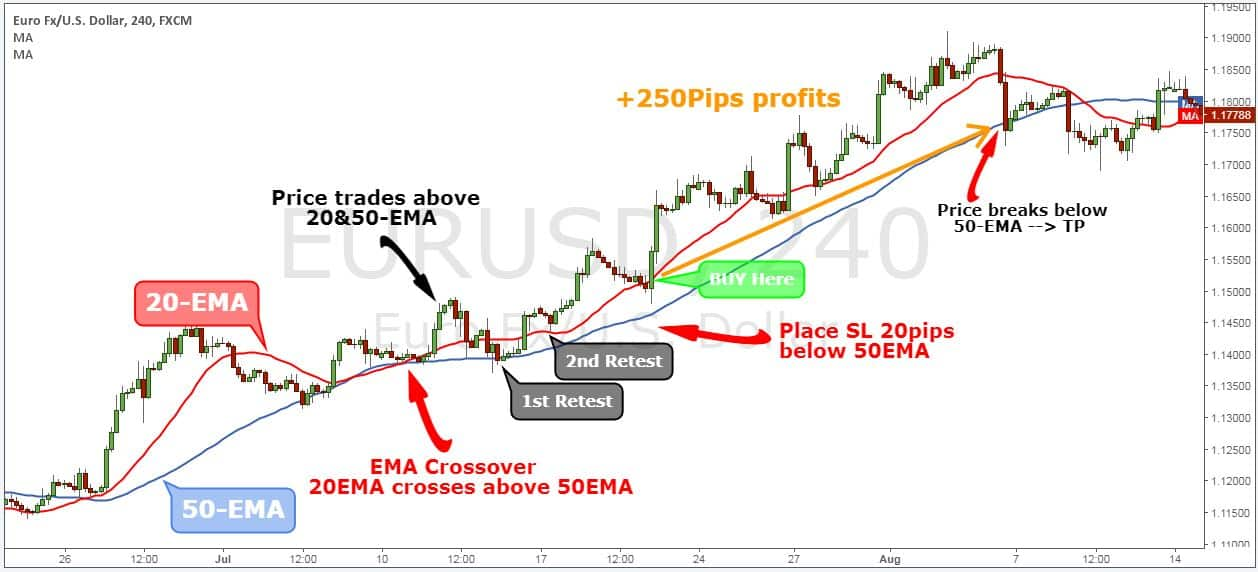Note** The above was an example of a BUY trade. Use the same rules – but in reverse – for a SELL trade. However, because the market goes down much faster, we sell on the 1st retest of the zone between 20 and 50. After the EMA crossover happened.

In the figure below, you can see an actual SELL trade example, using our strategy.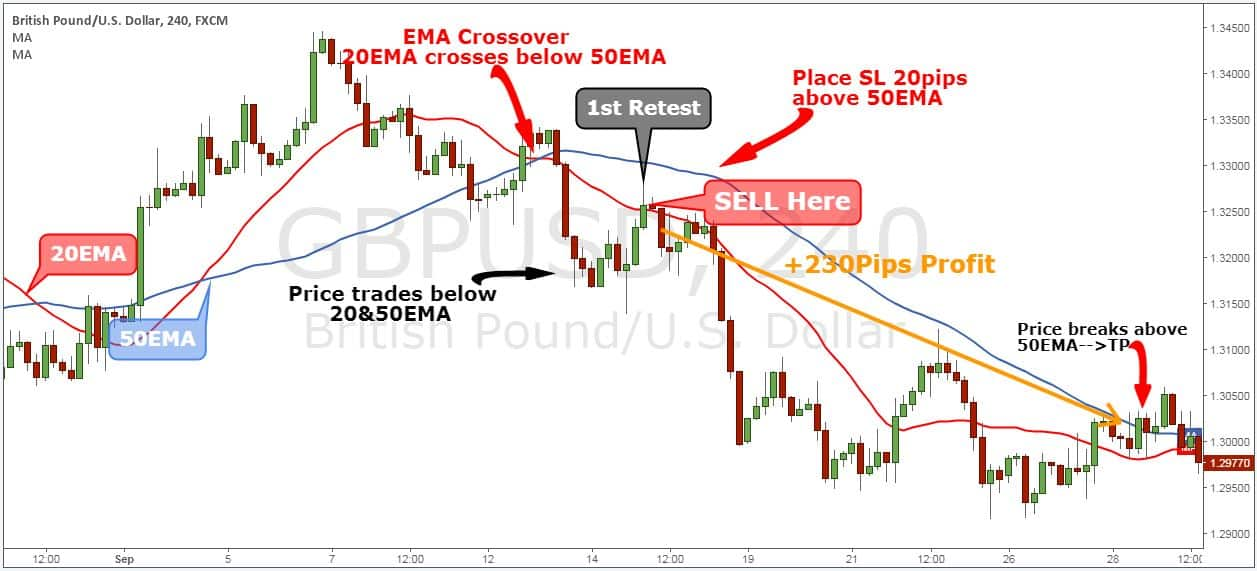### Summary

The exponential moving average strategy is a classic example of how to construct a simple EMA crossover system. With this exponential moving average system, we’re not trying to predict the market. We're trying to react to the current market condition, which is a much better way to trade.

The advantage of our trading strategy stands in the exponential moving average formula. It plots a much smoother EMA that gives better entries and exits.

We understand there are different trading styles. If following term trends are not for you, try reading our Best Short Term Trading Strategy – Profitable Short Term Trading Tips. It reveals a short-term trading trick used by institutional traders.